# How to Add a Boolean Datatype Column to an Existing Table in SQL?

• Last Updated : 21 Nov, 2021

In SQL Server, a Boolean Datatype can be created by means of keeping BIT datatype. Though it is a numeric datatype, it can accept either 0 or 1 or NULL values only. Hence easily we can assign FALSE values to 0 and TRUE values to 1. This will provide the boolean nature for a data type.

Regarding the storage, if there are less than 9 columns of the bit data are present in the table,  they are stored as 1 byte. And for 9 to 16 BIT columns,  their consumption is 2 bytes and so on it will hold. In this article, let us how we can add a Boolean i.e. BIT datatype to an existing table in SQL SERVER.

Let us assume there is a database “GEEKSFORGEEKS” is available and there is a table called “Authors” available with the following data.

Query:

```USE GEEKSFORGEEKS
SELECT * FROM Authors;```

Output: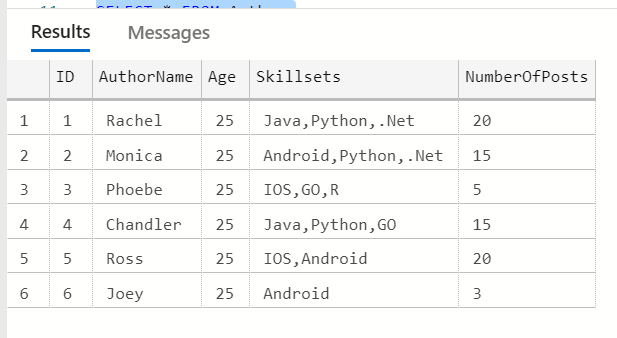Let us add a BIT data type to the “Authors” table. Bit datatype is helpful to represent Boolean nature either True(1) or False(0) and they are the only allowed values for BIT datatype.

```-- Add a column named "isActiveAuthor"
with BIT datatype for "Authors" table
ALTER TABLE Authors  ADD isActiveAuthor BIT;```

As there are already few rows available in the table, we can add a new column “isActiveAuthor” as a NULL pattern only. After that, we can update the data. After adding the column,

Query:

`SELECT * FROM Authors;`

Output: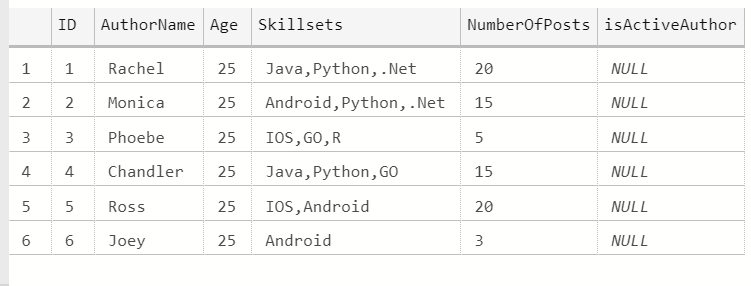So, when a BIT datatype column is added to an existing table, its value will be populated with “NULL” values.

Now let us try to update the column with a condition that if “NumberOfPosts” > 5, set “isActiveAuthor” to have the value 1 or else 0.

```-- Let us assume that if "NumberOfPosts"
is more than 5, author is active enough to write articles
-- Hence for this condition , set "isActiveAuthor"
column to have value 1
UPDATE Authors SET isActiveAuthor = 1 WHERE NumberOfPosts > 5;

-- On the other hand, if "NumberOfPosts"
is less  than and equal to 5,
-- author is not active in the recent days
and hence set "isActiveAuthor" column to have value 0
UPDATE Authors SET isActiveAuthor = 0 WHERE NumberOfPosts <= 5;

SELECT * FROM Authors;```

Output: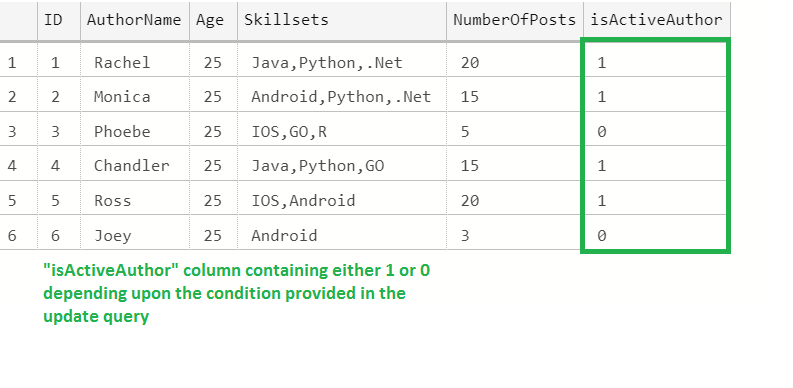As the BIT column supports only 0 or 1 as values, we have populated like above.

Let us try to update the value other than 0 or 1

```-- Trying to update the "isActiveAuthor"
with value 2, though errors are not
produced, output is different
UPDATE Authors SET isActiveAuthor =
2 WHERE NumberOfPosts = 5;

SELECT * FROM Authors;```

Output: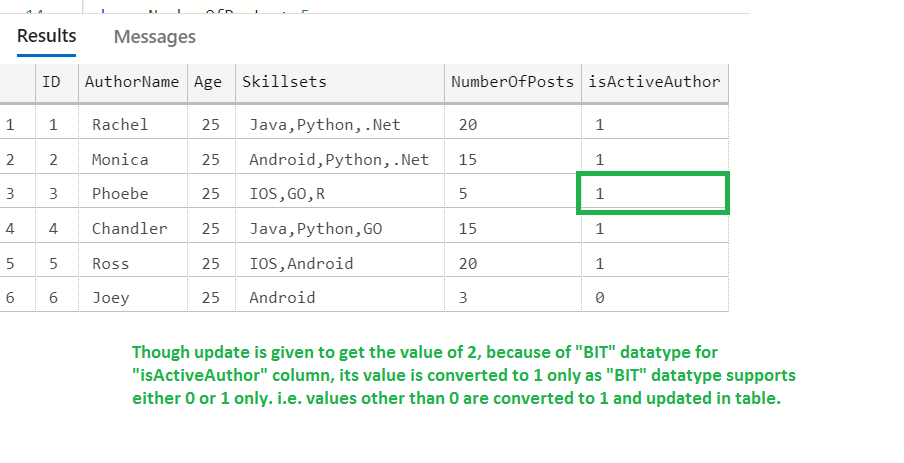Reason for the updated value of 1 in the 3rd row :

Though an update is given to get the value of 2, because of the “BIT” datatype for “isActiveAuthor” column, its value is converted to 1 only as the “BIT” datatype supports either 0 or 1 only. i.e. values other than 0 are converted to 1 and updated in the table.

We can check the same here with different examples

```DECLARE @isValid BIT

-- Initially it will have "NULL" value
SELECT @isValid AS BitType

--Assigning any nonzero value converts it to 1
-- i.e. other than 0, if any
value is provided , it is converted to 1
SET @isValid=9

SELECT @isValid AS BitType

--Assigning any nonzero value converts it to 1
SET @isValid=-100

SELECT @isValid AS BitType```

Output: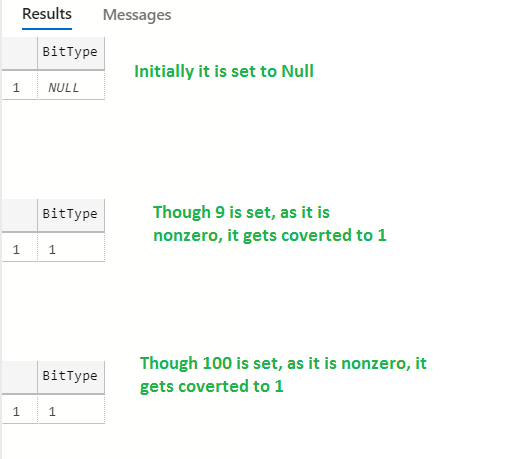This proves the “BIT” datatype accepts only the “Boolean” value i.e. either 0 or 1 only. If a nonzero value is given, it is converted to 1 only.